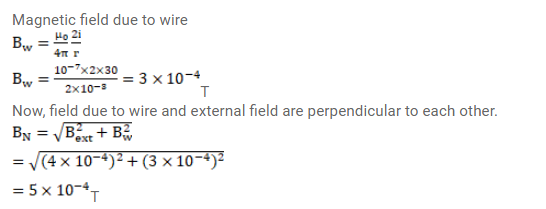# A long, straight wire carrying a current of

Question:

A long, straight wire carrying a current of $30 \mathrm{~A}$ is placed in an external, uniform magnetic field of $4.0 \times 10^{-4} \mathrm{~T}$ parallel to the current. Find the magnitude of the resultant magnetic field at a point $2.0 \mathrm{~cm}$ away from the wire.

Solution: